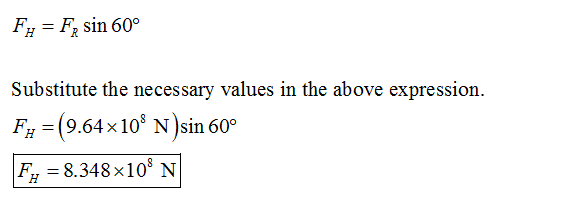# The water in a 25 m deep reservoir is kept inside by a 150 m wide wall whose cross section is an equilateral triangle. Determine a) the total hydrostatic force acting on the inner surface of the wall and its line of action, b) the magnitude of the horizontal component of this force.

Question-AnswerCategory: Fluid MechanicsThe water in a 25 m deep reservoir is kept inside by a 150 m wide wall whose cross section is an equilateral triangle. Determine a) the total hydrostatic force acting on the inner surface of the wall and its line of action, b) the magnitude of the horizontal component of this force.

The water in a 25 m deep reservoir is kept inside by a 150 m wide wall whose cross section is an equilateral triangle. Determine a) the total hydrostatic force acting on the inner surface of the wall and its line of action, b) the magnitude of the horizontal component of this force.

Step 1:
Consider hc as the hydrostatic center of the water, b is the length of the side at which the hydrostatic force, FR is the hydrostatic force, yb is the distance of the point of action of resultant force along the wall of reservoir and h is the height of water level. Draw the figure indicating all the forces acting on the reservoir as follows:Step 2

Write the trigonometric identity of sine  Î”ABC.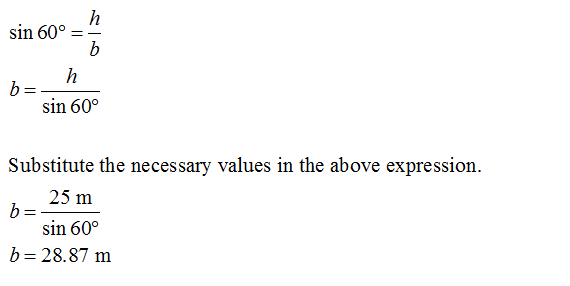Write the trigonometric identity of sine for Î”ADE.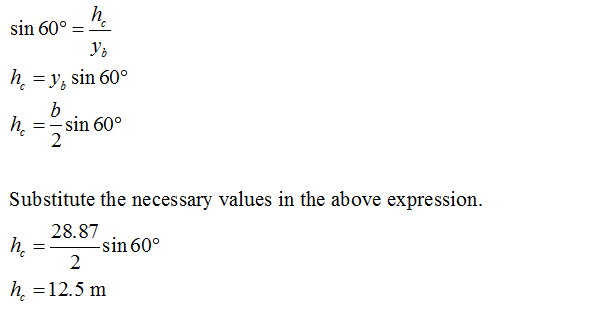Step 3

Write the expression of the resultant hydrostatic force.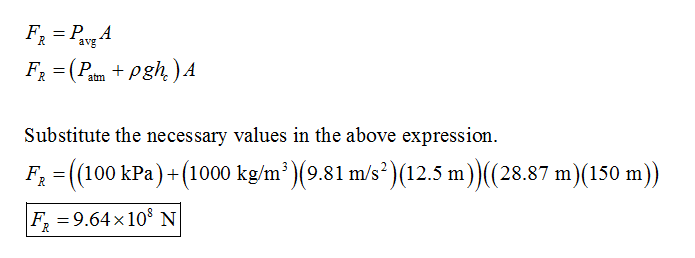Step 4

Understand that the reservoir is not fully submerged in water. Thus, s = 0. Write the expression of the distance of the point of action of resultant force along the wall of the reservoir.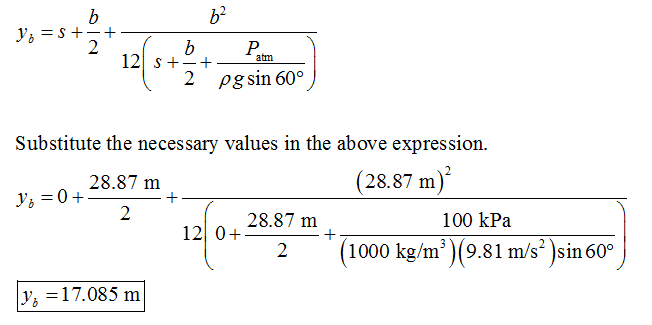Step 5 (b)

Consider FH as the horizontal force component of the hydrostatic force. Write the expression of the horizontal component of hydrostatic force.